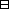## Group Theory and Linear Algebra

Last updated: 21 September 2014

## Lecture 9: Change of basis

Let $V$ be a vector space. Let $B=\left\{{b}_{1},{b}_{2},\dots \right\}$ be a basis of $V\text{.}$ Let $C=\left\{{c}_{1},{c}_{2},\dots \right\}$ be another basis of $V\text{.}$

The change of basis matrix from $B$ to $C$ is $P=(pij)given by cj=p1jb1 +p2jb2+⋯.$ The change of basis matrix from $C$ to $B$ is $Q=(qij)given by bj=q1jc1 +q2j+⋯.$ Let $f:V\to V$ be a linear transformation. The matrix of $f$ with respect to $B$ is $Bf=(fijB) given byf(bj)= f1jBb1+ f2jBb2+⋯.$ The matrix of $f$ with respect to $C$ is $Cf(fijC) given byf(cj)= f1jCc1+ f2jCc2+⋯.$

 (a) $P={Q}^{-1}\text{.}$ (b) ${B}_{f}=Q{C}_{f}{Q}^{-1}\text{.}$

My favourite vector space $V=ℂ3= { (a1a231) | a1,a2,a3∈ℂ }$ has basis $B={b1,b2,b3}$ with $b1=(100), b2=(010), b3=(001).$ The matrix $Bf= ( 001 100 010 )$ defines a linear transformation $f:V\to V,$ $f(b1)=b2, f(b2)=b3, f(b3)=b1$ and $f(3621) = f(3b1+6b2+21b3) = 3f(b1)+ 6f(b2)+ 21f(b3) = 3b2+6b3+21b1 = (2136).$ Another basis of $V$ is $C={c1,c2,c3}$ with $c1=(111), c2=(1-1+3i2-1-3i2), c2=(1-1-3i2-1+3i2).$ Then $c1 = b1+b2+b3, c2 = b1+(-1+3i2) b2+(-1-3i2)b3, c3 = b1+(-1-3i2) b2+(-1+3i2)b3,$ and $b1 = 13(c1+c2+c3), b2 = 13 ( c1+ -1-3i2c2+ -1+3i2c3 ) , b3 = 13 ( c1+ -1+3i2c2+ -1-3i2c3 ) .$ Helpful: Let $\zeta =\frac{-1+\sqrt{3}i}{2}$ and note that $ζ2 = (-1+3i2)2= 1-23i-34= -1-3i2and ζ3 = (-1-3i)2 (-1+3i)2= 1+34=1,$ and $1+ζ+ζ2=0.$ So $c1 = b1+b2+b3, c2 = b1+ζb2+ ζ2b3, c3 = b1+ζ2b2+ζb3$ and $13(c1+c2+c3) = 13 ( (111)+ (1ζζ2)+ (1ζ2ζ) ) =13(300)= (100)=b1, 13(c1+ζ2c2+ζc3) = 13 ( (111)+ ζ2(1ζζ2)+ ζ(1ζ2ζ) ) =13 ( (111)+ (ζ21ζ)+ (ζ1ζ2) ) =13(030)=b2, 13(c1+ζc2+ζ2c3) = 13 ( (111)+ ζ(1ζζ2)+ ζ2(1ζ2ζ) ) =13 ( (111)+ (ζζ21)+ (ζ2ζ1) ) =13(003)=bb.$ The change of basis matrix from $B$ to $C$ is $P= ( 111 1ζζ2 1ζ2ζ )$ and the change of basis matrix from $C$ to $B$ is $Q= ( 131313 1313ζ213ζ 1313ζ13ζ2 )$ and $PQ= ( 111 1ζζ2 1ζ2ζ ) ( 131313 1313ζ213ζ 1313ζ13ζ2 ) = ( 100 010 001 ) .$ So ${P}^{-1}=Q\text{.}$

The matrix of $f$ with respect to $C\text{:}$ $f(c1) = f(111) =(111) =c1, f(c2) = f(1ζζ2) =(ζ21ζ) =ζ2(1ζζ2) =ζ2c2, f(c3) = f(1ζ2ζ) =(ζ1ζ2) =ζ(1ζ2ζ) =ζc1.$ So the matrix of $f$ with respect to $C$ is $Cf= ( 100 0ζ20 00ζ ) .$ Magic: $QBfP = ( 131313 1313ζ213ζ 1313ζ13ζ2 ) ( 001 100 010 ) ( 111 1ζζ2 1ζ2ζ ) = 13 ( 111 1ζ2ζ 1ζζ2 ) ( 1ζ2ζ 111 1ζζ2 ) = 13 ( 100 03ζ20 003ζ ) = ( 100 0ζ20 00ζ ) = Cf.$

Let $f:V\to V$ be a linear transformation. Let $B$ and $C$ be bases of $V\text{.}$ Let $P$ be the change of basis matrix from $B$ to $C,$
$Q$ the change of basis matrix from $C$ to $B,$
${B}_{f}$ the matrix of $f$ with respect to $B,$
${C}_{f}$ the matrix of $f$ with respect to $C\text{.}$
Then

 (a) $P={Q}^{-1},$ (b) ${B}_{f}=Q{C}_{f}{Q}^{-1}\text{.}$Proof.

(a)
To show:
 (aa) $PQ=1,$ (ab) $QP=1\text{.}$
(aa)
We know: $P = (pij)with cj=p1j b1+p2jb2+⋯, Q = (qkℓ)with bℓ=q1ℓc1+ q2ℓc2+⋯.$
To show: $PQ=1\text{.}$
To show:
 (aaa) ${\left(PQ\right)}_{ii}=1,$ (aab) ${\left(PQ\right)}_{ij}=0$ if $i\ne j\text{.}$
 (aaa) $(PQ)ii= pi1q1i+ pi2q2i+ pi3q3i+⋯$ since $bi = q1ic1+ q2ic2+ q3ic3+⋯ = q1i ( p11b1+ p21b2+ p31b3+⋯ ) + q2i ( p12b1+ p22b2+ p32b3+⋯ ) + q3i ( p13b1+ p23b2+ p33b3+⋯ ) ⋮ = ( q1ip11+ q2ip12+ q3ip13+⋯ ) b1+ ( q1ip21+ q2ip22+ q3ip23+⋯ ) b2+⋯.$ If we use sum notation $bj = ∑ℓ qℓjcℓ = ∑ℓ qℓj ( ∑m pmℓbm ) = ∑ℓ,m pmℓ qℓjbm.$ So $∑ℓpmℓ qℓj=0$ if $m\ne j$ and $∑ℓpjℓ qℓj=1.$

$\square$

## Notes and References

These are a typed copy of Lecture 9 from a series of handwritten lecture notes for the class Group Theory and Linear Algebra given on August 12, 2011.